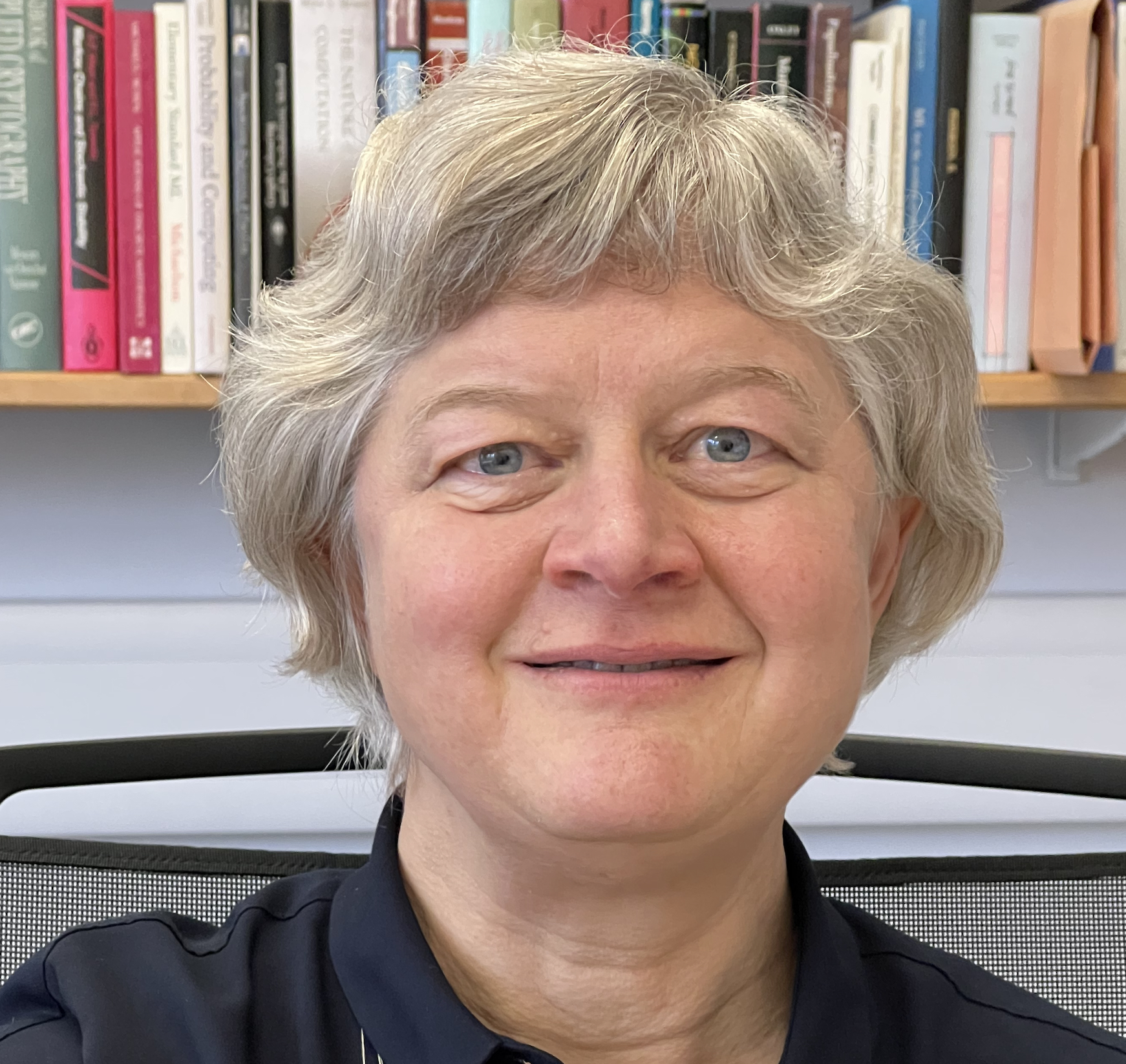• Professor Leslie Ann Goldberg

• Direct Email: leslie.goldberg@seh.ox.ac.uk
• Alternative Email: leslie.goldberg@cs.ox.ac.uk
• Office: 330 Wolfson Building

• Address: Department of Computer Science, University of Oxford,
Wolfson Bldg, Parks Rd,
Oxford OX1 3QD United Kingdom

I am a Professor of Computer Science and head of the Algorithms and Complexity Theory Reseach Theme at the University of Oxford and a Senior Research Fellow at St Edmund Hall.

I am on sabbatical through Sept 2021. When I return at the start of Oct 2021, I will serve as the Head of Department and will resume teaching the MSc and Part C course Probability and Computing.

## Research Interests

I am interested in the design and analysis of randomised algorithms and in the probabilistic analysis of algorithms. Many important randomised algorithms are based on simulating Markov chains and other stochastic processes. Key research questions include determining how long a Markov chain must be simulated before it reaches a distribution which is close to its stationary distribution, determining convergence rates of other stochastic processes, and using these convergence rates to obtain algorithmic performance guarantees. Examples of this work include

• Phase Transitions of the Moran Process and Algorithmic Consequences. The Moran process is a random process that models the spread of genetic mutations through graphs. In this work with John Lapinskas and David Richerby, we give an almost-tight bound on the expected time that the process takes to reach either "fixation", where every vertex is a mutant, or "extinction", where no vertex is a mutant. We use this to obtain an improved algorithm for approximating the probability of fixation.

"Spin systems" are statistical physics models in which there is an underlying graph and in each "configuration" every vertex is assigned a "spin" (which you can think of as a positive integer from some fixed range). The local interactions between the spins (caused by the edges of the graph) give rise to the global "energy", or weight, of a configuration. Computational problems including sampling configurations with probabilities proportional to their weights, and estimating the "partition function", which is the sum of the weights of all configurations. Here is a recent example of work applying Markov-chain techniques to these problems.

• Fast algorithms at low temperatures via Markov chains . Recently, models called "polymer models" have been useful in the development of good algorithms for sampling and estimating partition functions of spin systems. In this work with Zongchen Chen, Andreas Galanis, Will Perkins, James Stewart, and Eric Vigoda, we define a discrete-time Markov chain for abstract polymer models and show that, under sufficient decay of the polymer weights, this chain mixes rapidly. We use this to obtain fast algorithms for sampling configurations and estimating the partition function.

The problem of estimating the partition function of a spin system gives rise to foundational complexity-theoretic problems. Such a spin system is typically characterised by several parameters. For some values of the parameters, it is possible to build good sampling and estimation algorithms. For some other values of the parameters, it is possible to prove that such good algorithms do not exist, subject to complexity-theoretic conjectures similar to the famous "P not equal to NP" conjecture. This leads to a fundamental classification problem - determining for which parameter-values good algorithms exist, and for which there are provably no good algorithms. Examples of this work include

• Inapproximability of the independent set polynomial in the complex plane. This work with Ivona Bezakova, Andreas Galanis and Daniel Stefankovic provides an inapproximability result for the so-called independence polynomial of a graph, which is the same as the partition function of the hard-core model in statistical physics. An important feature of this work, and of other recent work in the area, is the role of complex parameter-values in determining the complexity of the problem.

The problem of estimating a partition fuctions can be viewed as a generalisation of a computational problem in which the goal is to count combinatorial structures. A key example is #BIS, the problem of counting the independent sets of a bipartite graph. The following paper with Martin Dyer, Catherine Greenhill and Mark Jerrum introduced this problem and showed that it is complete in a large complexity class for approximate counting.
We now know that #BIS is equivalent in difficulty to a large number of other computational problems, so it would be wonderful to resolve the question of whether it has a fast approximation algorithm.